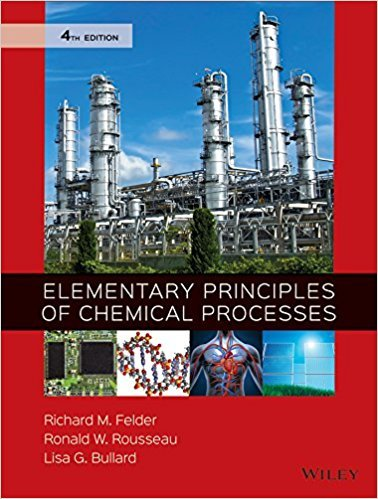×
×

# Solutions for Chapter 4.24: SUMMARY## Full solutions for Elementary Principles of Chemical Processes | 4th Edition

ISBN: 9780470616291Solutions for Chapter 4.24: SUMMARY

Solutions for Chapter 4.24
4 5 0 309 Reviews
30
1
##### ISBN: 9780470616291

This expansive textbook survival guide covers the following chapters and their solutions. This textbook survival guide was created for the textbook: Elementary Principles of Chemical Processes, edition: 4. Since 1 problems in chapter 4.24: SUMMARY have been answered, more than 45718 students have viewed full step-by-step solutions from this chapter. Chapter 4.24: SUMMARY includes 1 full step-by-step solutions. Elementary Principles of Chemical Processes was written by and is associated to the ISBN: 9780470616291.

Key Chemistry Terms and definitions covered in this textbook
• absorption spectrum

In IR spectroscopy as well as UV-VIS spectroscopy, a plot that measures the percent transmittance or absorption as a function of frequency.

• alkyl group

A group that is formed by removing a hydrogen atom from an alkane. (Section 25.3)

• alpha (a) rays.

Helium ions with a positive charge of 12. (2.2)

• angle strain

The increase in energy associated with a bond angle that has deviated from the preferred angle of 109.5°.

• atomic mass unit (amu).

A mass exactly equal to 1 12th the mass of one carbon-12 atom. (3.1)

• beta sheet

A structural form of protein in which two strands of amino acids are hydrogen-bonded together in a zipperlike configuration. (Section 24.7)

• bond polarity

A measure of the degree to which the electrons are shared unequally between two atoms in a chemical bond. (Section 8.4)

• cellulose

A polysaccharide of glucose; it is the major structural element in plant matter. (Section 24.8)

• Delocalization

The spreading of electron density over a larger volume of space.

• diamagnetic.

Repelled by a magnet; a diamagnetic substance contains only paired electrons. (7.8)

• donor atom

The atom of a ligand that bonds to the metal. (Section 23.2)

• functional group

An atom or group of atoms that imparts characteristic chemical properties to an organic compound. (Section 24.1)

• hydronium ion 1H3O+2

The predominant form of the proton in aqueous solution. (Section 16.2)

• intensive property

A property that is independent of the amount of material considered, for example, density. (Section 1.3)

• paramagnetism

A property that a substance possesses if it contains one or more unpaired electrons. A paramagnetic substance is drawn into a magnetic field. (Section 9.8)

• Planck constant (h)

The constant that relates the energy and frequency of a photon, E = hn. Its value is 6.626 * 10-34 J@s. (Section 6.2)

• polyurethanes

Polymers made up of repeating urethane groups, also sometimes called carbamate groups (!N!CO2!).

• protium

The most common isotope of hydrogen. (Section 22.2)

• solvolysis

A substitution reaction in which the solvent functions as the nucleophile.

• specific heat 1Cs2

The heat capacity of 1 g of a substance; the heat required to raise the temperature of 1 g of a substance by 1 °C. (Section 5.5)

×